# Re: Hornsub shootout RESULTS - Bassmaxx Z5

[ ProSpeakers Forum ] [ Help ]

Posted by Wayne Parham [ 67.66.231.14 ] on October 15, 2005 at 14:20:12:

In Reply to: Re: Hornsub shootout RESULTS posted by Wayne Parham on October 15, 2005 at 12:02:43:### Bassmaxx Z5 basshorn sub

The Bassmaxx Z5 was next in line. It weighs in at about 180 lbs and measures 42" x 42" x 22.5". It uses a single 18" Merlin driver.

Specifications:

Weight: 180 lbs
Dimensions: 42" x 42" x 22.5"
Power handling: 1200wrms
Impedance: 8Ω
Frequency range: 30Hz - 170Hz
Sensitivity: 105dB/W/M
Max Output: 133dB/M
Distortion at 100 watts: 2%

First, we measured impedance and found Zmin to be 8.0Ω. So 28.28v is required for 100 watts, 40v for 200 watts, 56.56v for 400 watts, 80v for 800 watts and 98v for 1200 watts. We joked that max power is just about right to run your home. Just plug these things into the wall socket.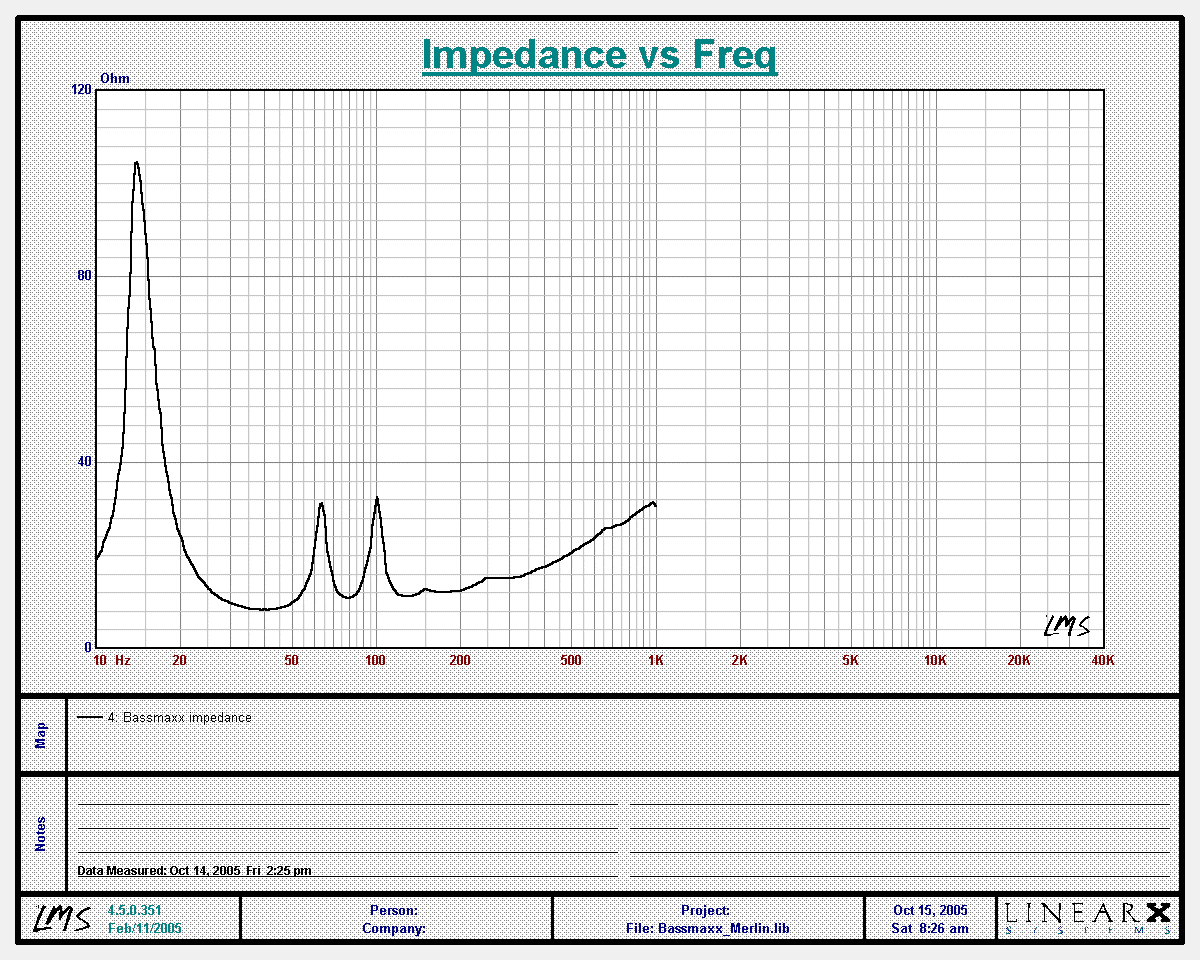### Bassmaxx impedance

Next, we measured output at 28.28v. This generates 100 watts. Since we measured 10 meters away, the values correspond to both 2.83v/1M and 1W/1M.

The blue line is SPL, and the violet line shows distortion. Average distortion is 35dB under the fundamental, which is about 2%. Since measurement was taken at 10 meters, add 20dB to find SPL at 1 meter. With 100 watts input, the 10 meter measurement works out the same as 1 watt input measured at a distance of 1 meter.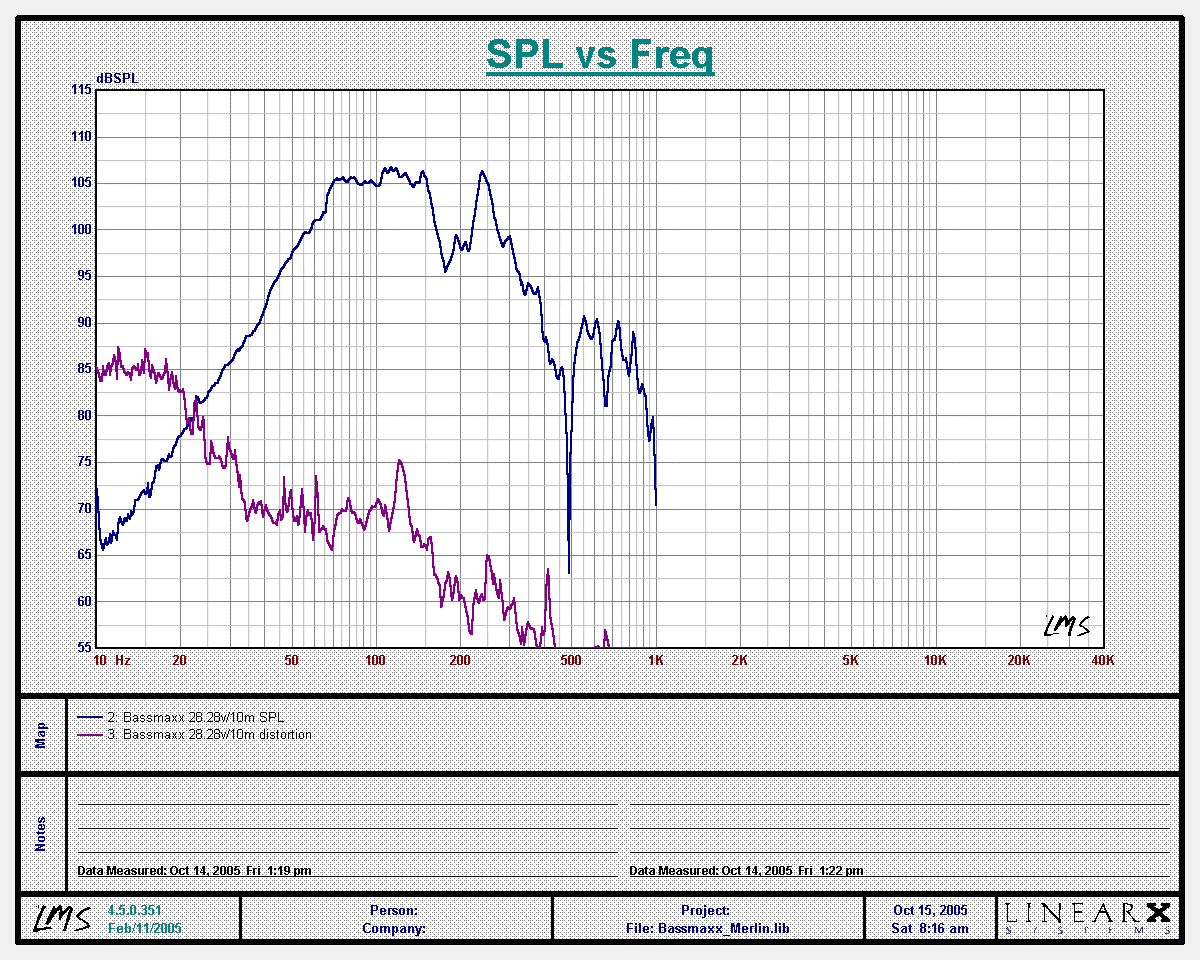### Bassmaxx response at 10 meters with 28.28v input (2.83v/1M)

From this, we perform an inverse FFT to obtain impulse and step response: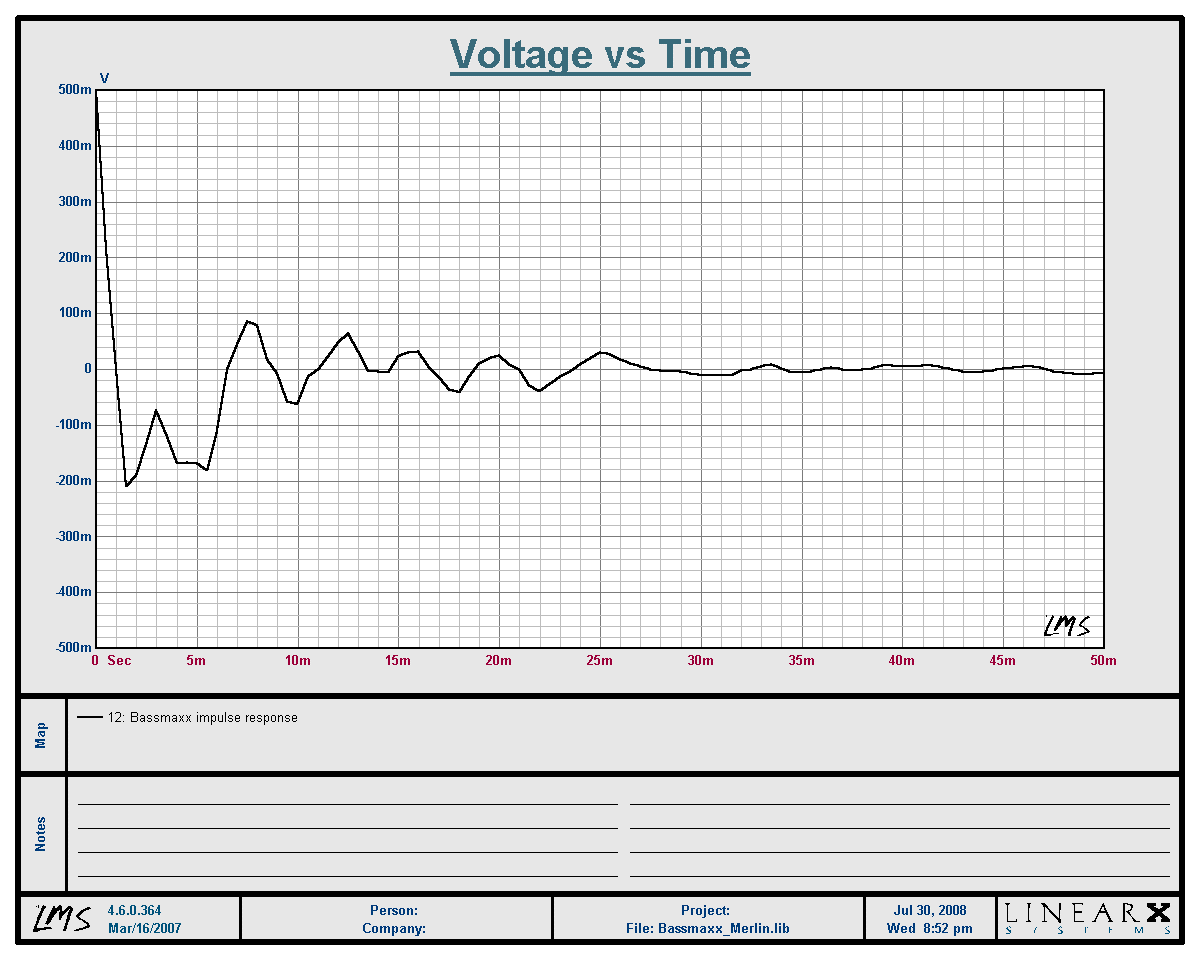### Bassmaxx Impulse Response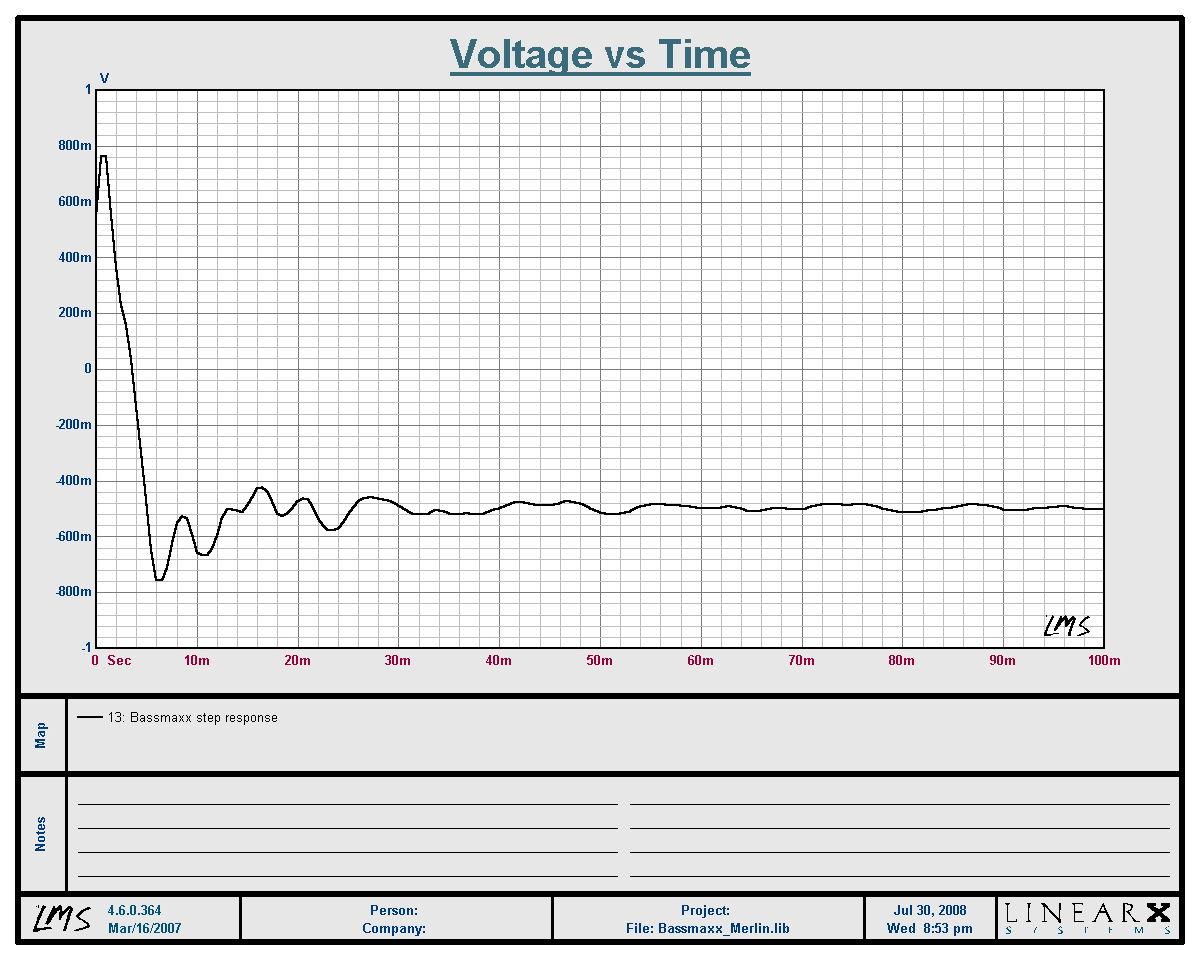### Bassmaxx Step Response

Measurement at 200 watts: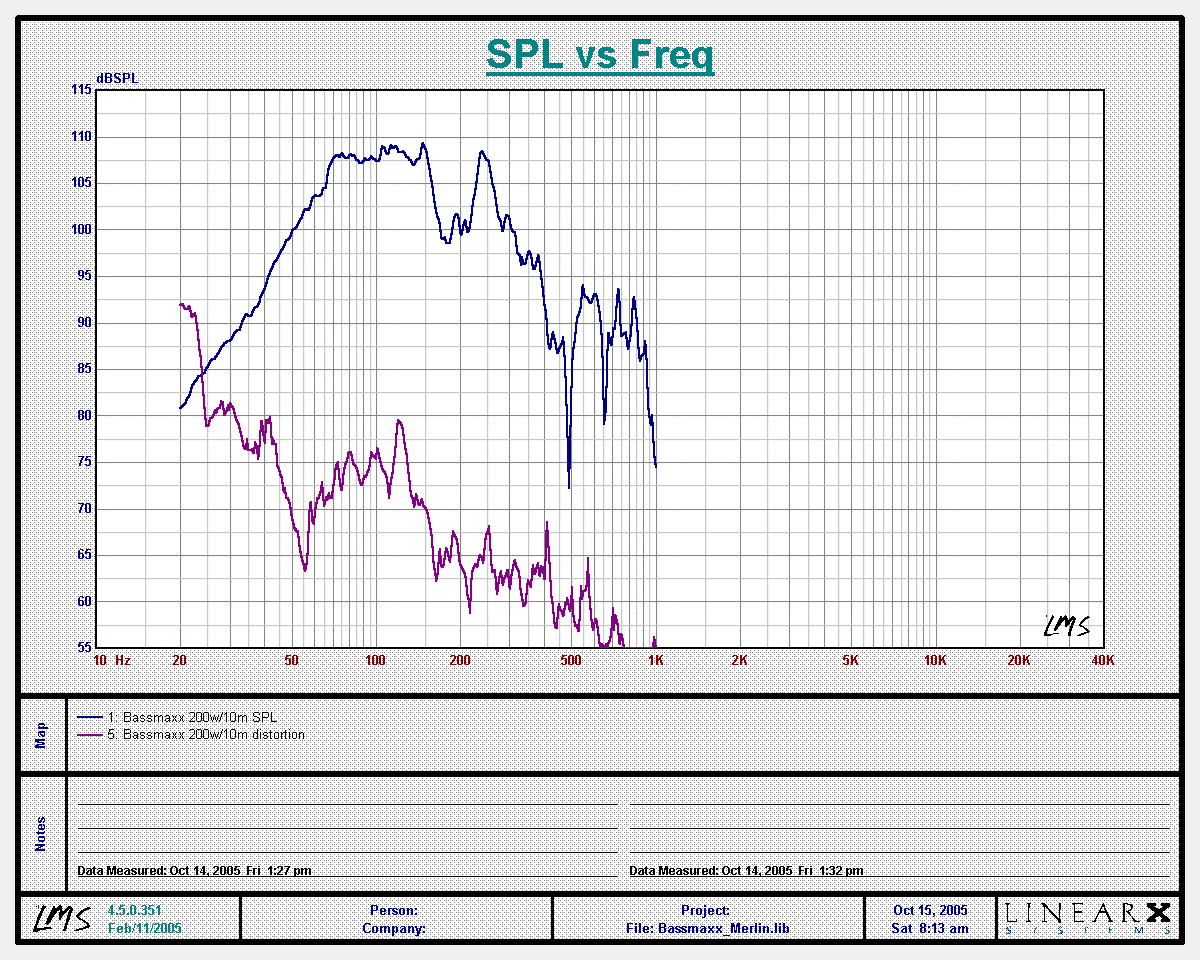### Bassmaxx response at 10 meters with 40v (200w) input

Output at 200 watts measured at 10 meters is about 108dB average between 70Hz and 150Hz, which is 128db at 1 meter.

Measurement at 400 watts:### Bassmaxx response at 10 meters with 56.56v (400w) input

Output at 400 watts measured at 10 meters is about 110dB average between 70Hz and 150Hz, which is 130db at 1 meter.

Measurement at 800 watts: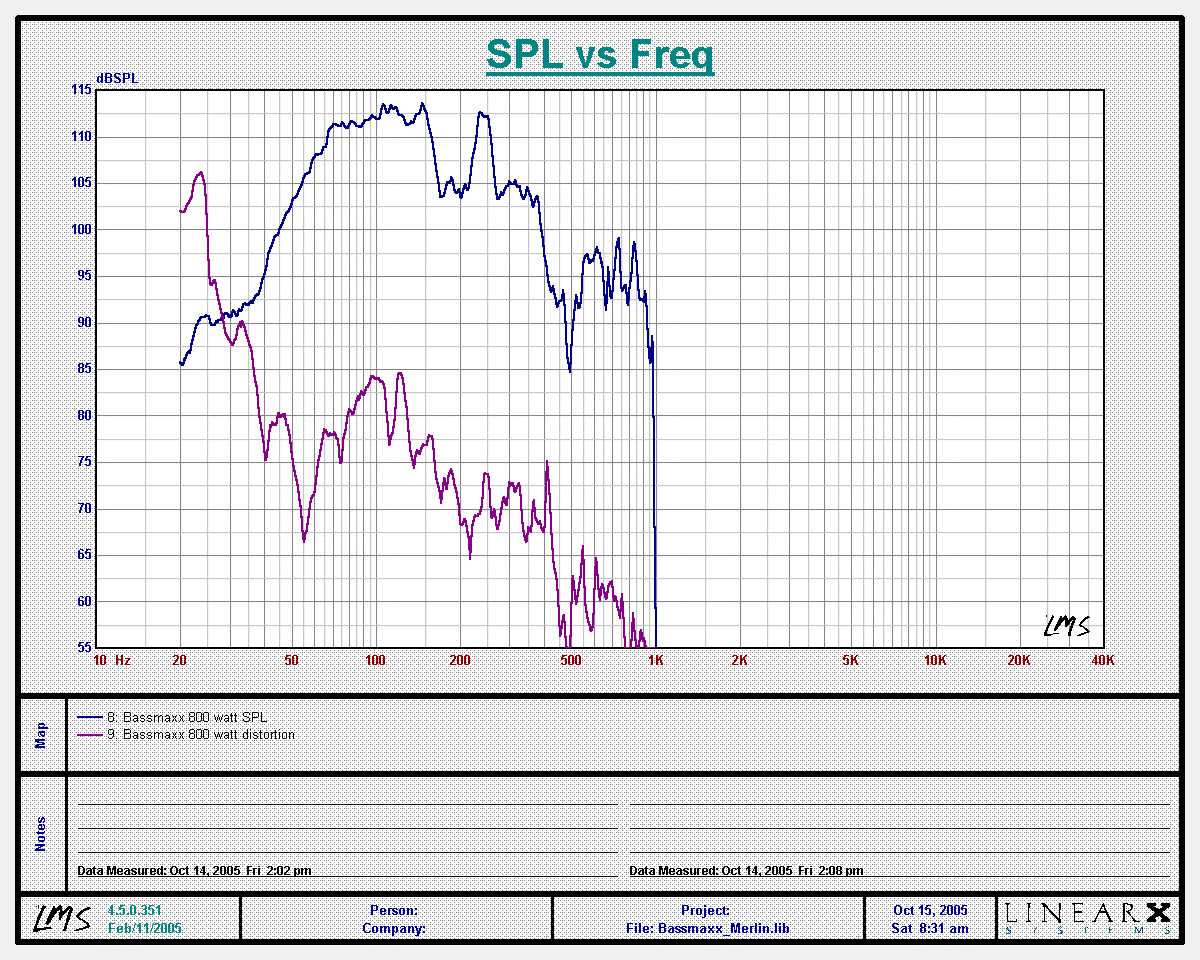### Bassmaxx response at 10 meters with 80v (800w) input

Output at 800 watts measured at 10 meters is about 112dB average between 70Hz and 150Hz, which is 132db at 1 meter.### Bassmaxx response at 10 meters with 98v (1200w) input

Output at 1200 watts measured at 10 meters is about 113dB average between 70Hz and 150Hz, which is 133db at 1 meter.

[ ProSpeakers Forum ] [ Help ]